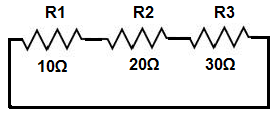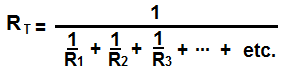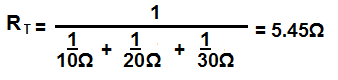﻿ Resistors in Series and in Parallel ﻿# Resistors in Series and in ParallelWe will go over the mathematical formulas for calculating series and parallel resistance so that we can compute the total resistance values of actual circuits.

### Resistors in Series

Resistors in series are resistors that are placed back-to-back.

Below is a circuit where 3 resistors are placed in series.You can see the resistors are in series because they are back-to-back against each other. The best way to think of a series circuit is that if current flows through the circuit, the current can only take one path. You can see in the above circuit that if current flowed through it, it could only take one path.

### Formula for Adding Resistors in Series

The formula to calculate the total series resistance of a circuit is:So to calculate the total resistance of the circuit above, the total resistance, RT would be:So using the above formula, the total resistance is 60Ω.

Note- When resistors are in series, as the formula shows, they simply add together. Thus, the total resistance of a series circuit will always be greater than any of the individual resistor values.

### Resistors in Parallel

Resistors in parallel are resistors that are connected side-by-side in different branches of a circuit.

Below is a circuit where 3 resistors are in parallel:You can see that the resistors are in parallel because they are all on their own separate branches in the circuit. The best way to think about parallel circuits is by thinking of the path that current can take. When current is travelling through a parallel circuit, the current can take various paths through the circuits, such as to go through any of the branches of the resistors. In series, this is not the case. Current can only take one path.

### Formula for Adding Capacitors in Parallel

The formula to calculate the total parallel capacitance is:So to calculate the total resistance of the circuit above, the total resistance, RT would be:So using the above formula, the total resistance is 5.45Ω.

Note- When resistors are in parallel, the total resistance value is always less than the smallest resistor of the circuit. In other words, when resistors are in parallel, the total resistance shrinks. It's always less than any of the values of the resistors. The resistance doesn't increase in parallel; it decreases.

### Resistor Circuit in Series and In Parallel

We'll now do a resistor circuit in which resistors are both in series and in parallel in the same circuit.

Below is a circuit which has resistors in both series and parallel:So how do we add them to find the total resistance value?

First, we can start by finding the resistance of the resistors in series. In the first branch, containing the 20Ω and 40Ω resistors, the series resistance is 60Ω. And in the second branch, containing the 30Ω and 60Ω resistors, the series resistance is 90Ω. Now in total, the circuit has 3 resistances in parallel, 10Ω, 60Ω, and 90Ω. Now, we plug these 3 values into the parallel resistance calculator and get a total resistance of 7.83Ω.

If you want to test the above series and parallel connections out practically, get 1KΩ resistor or whatever resistors you have, but let them be of the same value. In this example, I'll stick with 2 1KΩ resistors. Take the resistors and place them in series. Now take a multimeter and place the multimeter in the ohmmeter setting and place the probes over the 2 resistors You should read just about 2KΩ, which is double the value of both resistors. This proves that resistors add when connected in series. Now place the resistors in parallel. Take the multimeter probes and place one end on one side of a resistor (either one) and place the other probe on the other side of that resistor. You should now read about 0.5KΩ, or half the value, because resistance in parallel decreases. This is a practical, real-life test you can do to show how resistors add.

Related Resources